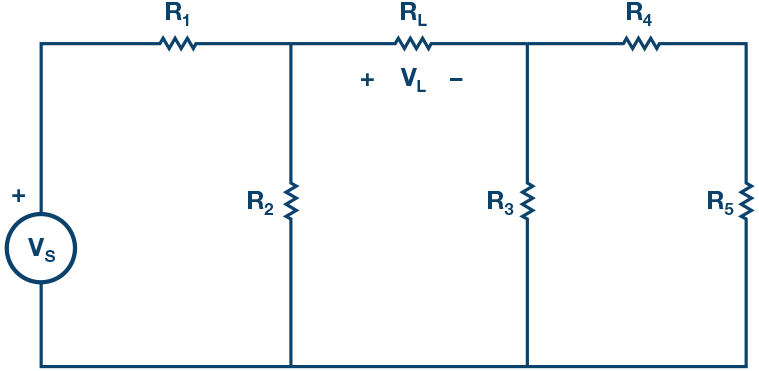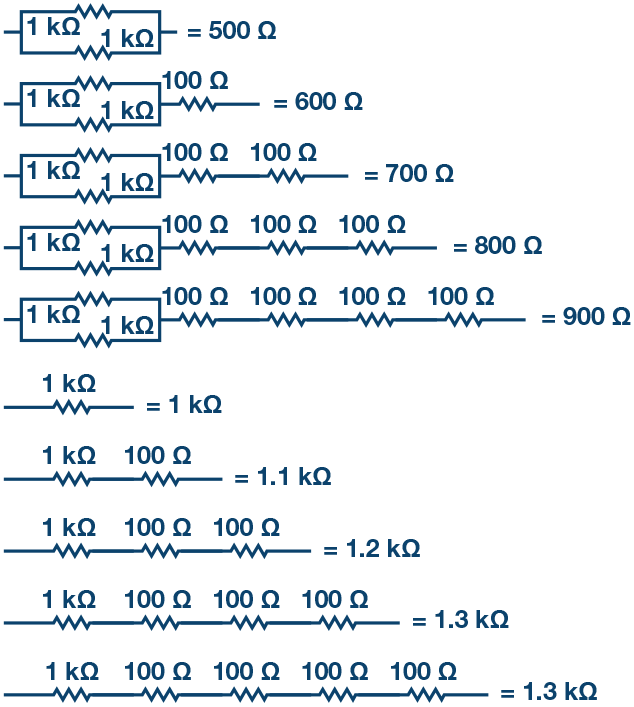# ADALM1000 SMU Training Topic 3: Thévenin Equivalent Circuit and Maximum Power Transfer

After the introduction of the SMU ADALM1000 in our Analog Dialogue December 2017 article, we want to continue with the third part of our ADALM1000 series with some small, basic measurements. You can find the first ADALM1000 article here.Figure 1. A schematic of the ADALM1000.

Now let’s get started with the next experiment.

### Objective

The objective of this lab activity is to verify Thévenin’s theorem by obtaining the Thévenin equivalent voltage (VTH) and Thévenin equivalent resistance (RTH) for the given circuit, and then to verify the maximum power transfer theorem.

### Background

Thévenin’s theorem is a process by which a complex circuit is reduced to an equivalent circuit consisting of a single voltage source (VTH) in series with a single resistance (RTH) and a load resistance (RL). After creating the Thévenin equivalent circuit, the load voltage VL or the load current IL may be easily determined.

One of the principal uses of Thévenin’s theorem is to replace a large portion of a circuit, often a more complicated and uninteresting part, with a simple equivalent. The new simpler circuit enables rapid calculations of the voltage, current, and power than the more complicated original circuit is able to deliver to a load. The theorem also helps to choose the optimal value of the load (resistance) for maximum power transfer.Figure 2. Thévenin equivalent circuit of Figure 1.

The maximum power transfer theorem states that an independent voltage source in series with a resistance, RS, or an independent current source in parallel with a resistance RS delivers a maximum power to the load resistance, RL, when RL = RS.

In terms of a Thévenin equivalent circuit, maximum power is delivered to the load resistance RL when RL is equal to the Thévenin equivalent resistance, RTH, of the circuit.Figure 3. Maximum power transfer.

### Materials

• ADALM1000 hardware module
• Various resistors (100 Ω, 330 Ω, 470 Ω, 1 kΩ, and 1.5 kΩ)

### Procedure

1. Verifying Thévenin’s theorem:

1. Construct the circuit of Figure 2 using the following component values:

• R1 = 330 Ω
• R2 = 470 Ω
• R3 = 470 Ω
• R4 = 330 Ω
• R5 = 1 kΩ
• RL = 1.5 kΩ
• RS = 5 V
2. Accurately measure the voltage VL across the load resistance using the ALM1000 voltmeter tool. Use the voltmeter tool by connecting channel CA to the positive node of VL and connect channel CB to the negative node. VL will be the difference between CA volts and CB volts. This value will later be compared to the one you will find using the Thévenin equivalent.

3. Find VTH: Remove the load resistance, RL, and measure the open circuit voltage, VOC, across the terminals. Use the voltmeter tool by connecting channel CA to the positive node of VOC and connect channel CB to the negative node. VOC will be the difference between CA volts and CB volts. This is equal to VTH. See Figure 4.Figure 4. Measuring the Thévenin voltage.
4. Find RTH: Remove the source voltage VS and construct the circuit as shown in Figure 5. Use the ALM1000 ohmmeter tool to measure the resistance looking into the opening where RL was. This gives RTH. Make sure there is no power applied to the circuit before measuring with the ohmmeter and that the ground connection has been moved as shown.Figure 5. Measuring the Thévenin resistance, RTH.
5. Obtaining VTH and RTH, construct the circuit of Figure 2. Create the value of RTH using a series and or parallel combination of resistors from your parts kit. Using the meter-source tool, connect channel CA for the VTH source and set the value to what you measured for VTH in Step c.Figure 6. Thévenin equivalent construction.
6. With RL set to the 1.5 kΩ used in Step b, measure the VL for the equivalent circuit and compare it to the VL obtained in Step b. This verifies the Thévenin theorem.

7. Optional: repeat Steps 1b to Step 1f for RL = 2.2 kΩ.

2. Verifying the maximum power transfer theorem:

1. Construct the circuit as in Figure 7 using the following values:

• VS = 5 V
• R1 = R2 = 470 Ω
• R3 = 1 kΩ
• RL = combinations of 1 kΩ and 100 Ω resistors (Figure 8)Figure 7. Circuit for maximum power theorem.
2. Use the voltmeter tool by connecting channel CA to the positive node of VL and connect channel CB to the negative node across RL. VL will be the difference between CA volts and CB volts.

3. To find the value of RL for which maximum power is transferred, vary the load resistances by constructing series/parallel combinations of 1 kΩ and 100 Ω for RL between 500 Ω to 1400 Ω in 100 Ω steps, as shown in Figure 8. For each value of RL, write down VL.Figure 8. RL configurations.
4. Calculate the power for each load resistor value using PL = VL2/RL. Then, interpolate between your measurements to calculate the load resistor value corresponding to the maximum power (PL-max). This value should be equal to RTH of circuit in Figure 7 with respect to load terminals.

### Questions

1. Using voltage division for the circuit of Figure 2, calculate VL. Compare it to the measured values. Explain any differences.
2. Calculate the maximum power transmitted to the load RL obtained for the circuit of Figure 3.

You can find the answers at the StudentZone blog.

### Notes

As in all the ALM labs, we use the following terminology when referring to the connections to the ALM1000 connector and configuring the hardware. The green shaded rectangles indicate connections to the ADALM1000 analog I/O connector. The analog I/O channel pins are referred to as CA and CB. When configured to force voltage/measure current, –V is added (as in CA-V) or when configured to force current/measure voltage, –I is added (as in CA-I). When a channel is configured in the high impedance mode to only measure voltage, –H is added (as in CA-H).

Scope traces are similarly referred to by channel and voltage/current, such as CA-V and CB-V, for the voltage waveforms, and CA-I and CB-I for the current waveforms.

We are using the ALICE rev 1.1 software for those examples here.

File: alice-desktop-1.1-setup.zip. Please download here.

The ALICE desktop software provides the following functions:

• A 2-channel oscilloscope for time domain display and analysis of voltage and current waveforms.
• The 2-channel arbitrary waveform generator (AWG) controls.
• The X and Y display for plotting captured voltage and current vs. voltage and current data, as well as voltage waveform histograms.
• The 2-channel spectrum analyzer for frequency domain display and analysis of voltage waveforms.
• The Bode plotter and network analyzer with built-in sweep generator.
• An impedance analyzer for analyzing complex RLC networks and as an RLC meter and vector voltmeter.
• A dc ohmmeter measures unknown resistance with respect to known external resistor or known internal 50 Ω.
• Board self-calibration using the AD584 precision 2.5 V reference from the ADALP2000 analog parts kit.
• ALICE M1K voltmeter.
• ALICE M1K meter source.
• ALICE M1K desktop tool.

For more information, please look here.

Note: You need to have the ADALM1000 connected to your PC to use the software.